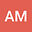loading page

Derivative of Unitary is not always -iHU
•• A S Muddu
A S Muddu
Reliance Corporate Park, Reliance Industries Limited, Mumbai 400701, India
Author Profile## Abstract

$i\dot{U} = HU$ (or $\dot{U} = -iHU$) is the equation that is  said to govern the evolution of a unitary matrix $U$ given the Hamiltonian $H$ of the system. This equation is said to hold true even if the Hamiltonian is time dependent. We show iU = HU (or $\dot{U} = -iHU$) is the equation that is  said to govern the evolution of a unitary matrix $U$ given the Hamiltonian $H$ of the system. This equation is said to hold true even if the Hamiltonian is time dependent. We show in this paper that $i\dot{U} = HU$ may not  always hold for time dependent Hamiltonians.this paper that $i\dot{U} = HU$ may not  always hold for time dependent Hamiltonians.